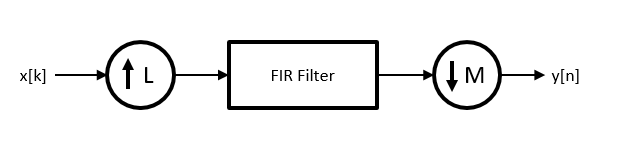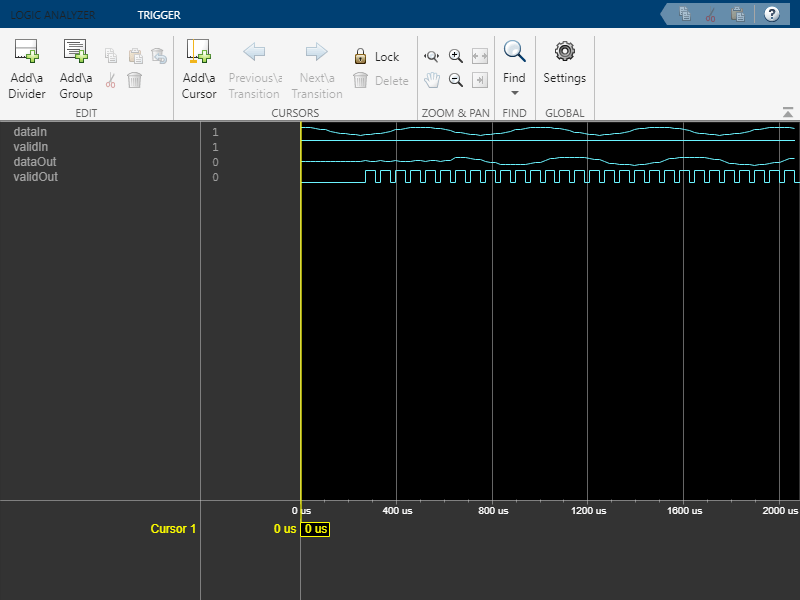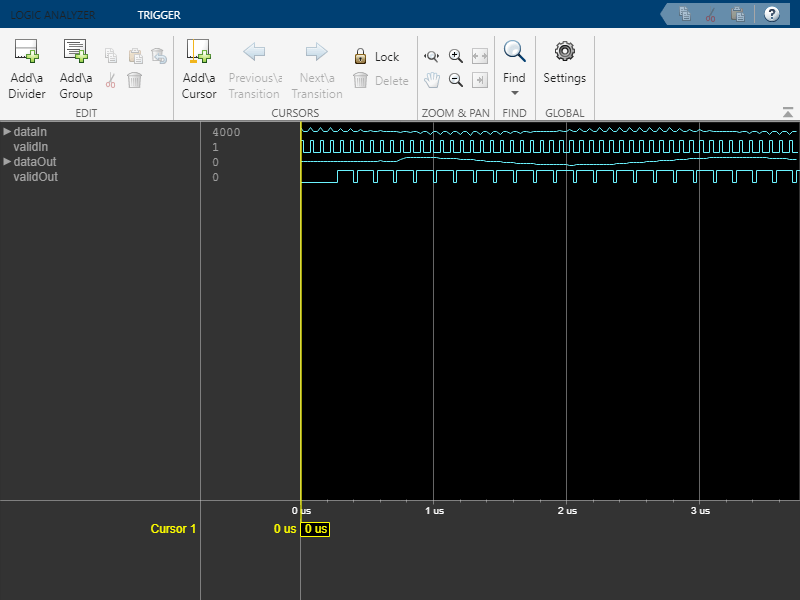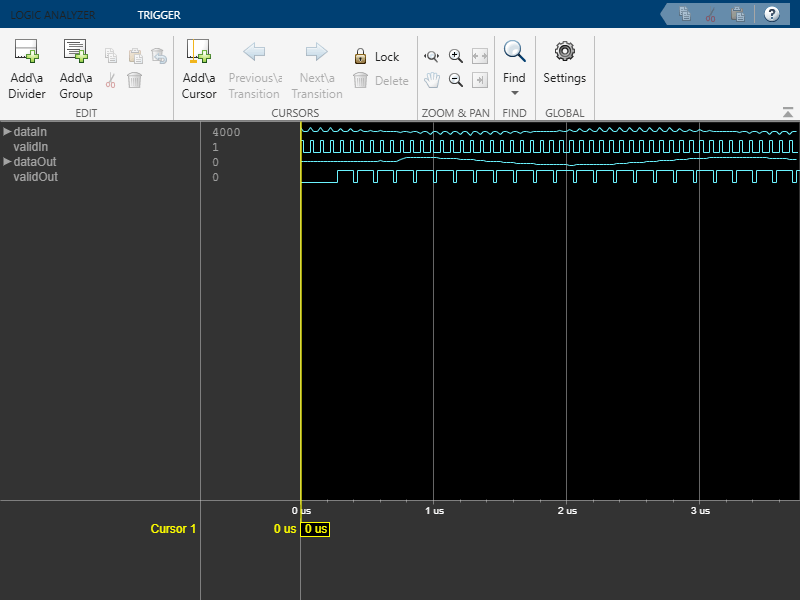# dsphdl.FIRRateConverter

Upsample, filter, and downsample input signal

## Description

The `dsphdl.FIRRateConverter` System object™ upsamples, filters, and downsamples input signals. It is optimized for HDL code generation and operates on one sample of each channel at a time. The object implements an efficient polyphase architecture to avoid unnecessary arithmetic operations and high intermediate sample rates.The object upsamples by an integer factor of L, applies an FIR filter, and downsamples by an integer factor of M.

To resample and filter input data:

1. Create the `dsphdl.FIRRateConverter` object and set its properties.

2. Call the object with arguments, as if it were a function.

## Creation

### Syntax

``HDLFIRRC = dsphdl.FIRRateConverter``
``HDLFIRRC = dsphdl.FIRRateConverter(L,M,num)``
``HDLFIRRC = dsphdl.FIRRateConverter(___,Name,Value)``

### Description

````HDLFIRRC = dsphdl.FIRRateConverter` returns a System object, `HDLFIRRC`, that resamples each channel of the input. The object upsamples by an integer factor of L, applies an FIR filter, and downsamples by an integer factor of M. The default L/M is 3/2.```

example

````HDLFIRRC = dsphdl.FIRRateConverter(L,M,num)` sets the `InterpolationFactor` property to `L`, the `DecimationFactor` property to `M`, and the `Numerator` property to `num`. ```
````HDLFIRRC = dsphdl.FIRRateConverter(___,Name,Value)` sets properties using one or more name-value pairs. Enclose each property name in single quotes. For example:HDLFIRRC = dsphdl.FIRRateConverter(L,M,Num,'ReadyPort',true);```

## Properties

expand all

Unless otherwise indicated, properties are nontunable, which means you cannot change their values after calling the object. Objects lock when you call them, and the `release` function unlocks them.

If a property is tunable, you can change its value at any time.

Upsampling factor, L, specified as a positive integer.

Downsampling factor, M, specified as a positive integer scalar.

FIR filter coefficients, specified as a vector in descending powers of z-1.

You can generate filter coefficients by using the Signal Processing Toolbox™ filter design functions, such as `fir1`. Design a lowpass filter with normalized cutoff frequency no greater than `min(1/L,1/M)`. The object initializes internal filter states to zero.

Enable `ready` output argument of the object. When enabled, the object returns a logical scalar value, `ready`, when you call the object. When `ready` is `1` (true), the object is ready for a new input sample the next time you call it.

Rounding mode used for fixed-point operations. This property does not apply when the input is `single` or `double` type. `'Simplest'` mode is not supported.

Overflow mode used for fixed-point operations. This property does not apply when the input is `single` or `double` type.

Data type of the FIR filter coefficients, specified as a `numerictype(s,wl,fl)` object with `signedness`, `word length`, and `fractional length` properties.

Data type of the output data samples, specified as '```Same word length as input```', '`Full precision`', or as a `numerictype(s,wl,fl)` object with `signedness`, `word length`, and `fractional length` properties.

## Usage

### Syntax

``````[dataOut,validOut] = HDLFIRRC(dataIn,validIn)``````
``````[dataOut,validOut,ready] = HDLFIRRC(dataIn,validIn)``````

### Description

example

``````[dataOut,validOut] = HDLFIRRC(dataIn,validIn)``` resamples `dataIn` according to the `InterpolationFactor` (L) and `DecimationFactor` (M) properties. To avoid dropped samples when using this syntax, apply new valid input samples, with `validIn` set to `true`, only every `ceil(L/M)` calls to the object. The object sets `validOut` to `true` when `dataOut` is a new valid sample.```

example

``````[dataOut,validOut,ready] = HDLFIRRC(dataIn,validIn)``` resamples the input data and returns `ready` to indicate whether the object can accept a new sample on the next call. This syntax applies when you set the `ReadyPort` property to `true`. For example: HDLFIRRC = dsphdl.FIRRateConverter(...,'ReadyPort',true); ... [dataOut,validOut,ready] = rateConverter(dataIn,validIn);```

### Input Arguments

expand all

Data input, specified as a scalar, or as a row vector where each element represents an independent channel.

`double` and `single` data types are supported for simulation, but not for HDL code generation.

Data Types: `fi` | `int8` | `int16` | `int32` | `int64` | `uint8` | `uint16` | `uint32` | `single` | `double`
Complex Number Support: Yes

Control signal that indicates if the input data is valid. When `validIn` is `1` (`true`), the object captures the values from the `dataIn` argument. When `validIn` is `0` (`false`), the object ignores the values from the `dataIn` argument.

You can apply a valid data sample every `ceil(L/M)` calls to the object. You can use the optional `ready` output signal to indicate when the object can accept a new sample.

Data Types: `logical`

### Output Arguments

expand all

Resampled and filtered data sample, returned as a scalar, or as a vector in which each element represents an independent channel.

`double` and `single` data types are supported for simulation, but not for HDL code generation.

Data Types: `fi` | `int8` | `int16` | `int32` | `int64` | `uint8` | `uint16` | `uint32` | `single` | `double`
Complex Number Support: Yes

Control signal that indicates if the output data is valid. When `validOut` is `1` (`true`), the object returns valid data from the `dataOut` argument. When `validOut` is `0` (`false`), values from the `dataOut` argument are not valid.

Data Types: `logical`

Control signal that indicates that the object is ready for new input data sample on the next cycle. When `ready` is `1` (`true`), you can specify the `data` and `valid ` inputs for the next time step. When `ready` is `0` (`false`), the object ignores any input data in the next time step.

#### Dependencies

To enable this argument, set the `ReadyPort` property to `true`.

Data Types: `logical`

## Object Functions

To use an object function, specify the System object as the first input argument. For example, to release system resources of a System object named `obj`, use this syntax:

`release(obj)`

expand all

 `step` Run System object algorithm `release` Release resources and allow changes to System object property values and input characteristics `reset` Reset internal states of System object

## Examples

collapse all

Convert a signal from 48 kHz to 32 kHz by using the `dsphdl.FIRRateConverter` System object™.

Define the sample rate and length of the input signal, and a 2 kHz cosine waveform. Set `validIn` = `true` for every sample.

```Fs = 48e3; Ns = 100; t = (0:Ns-1).'/Fs; dataIn = cos(2*pi*2e3*t); validIn = true(Ns,1);```

Preallocate `dataOut` and `validOut` signals for faster simulation.

```dataOut = zeros(Ns,1); validOut = false(Ns,1);```

Create the System object. Configure it to perform rate conversion by a factor of 2/3, using an equiripple filter.

```Numerator = firpm(70,[0 0.25 0.32 1],[1 1 0 0]); firrc = dsphdl.FIRRateConverter(2,3,Numerator);```

Call the System object to perform the rate conversion and obtain each output sample.

```for k = 1:Ns [dataOut(k),validOut(k)] = firrc(dataIn(k),validIn(k)); end```

Because the input sample rate is higher than the output sample rate, not every member of `dataOut` is valid. Use `validOut` to extract the valid samples from `dataOut`.

`y = dataOut(validOut);`

View the input and output signals with the Logic Analyzer.

```la = dsp.LogicAnalyzer('NumInputPorts',4,'SampleTime',1/Fs,'TimeSpan',Ns/Fs); modifyDisplayChannel(la,1,'Name','dataIn','Format','Analog','Height',8) modifyDisplayChannel(la,2,'Name','validIn') modifyDisplayChannel(la,3,'Name','dataOut','Format','Analog','Height',8) modifyDisplayChannel(la,4,'Name','validOut') la(dataIn,validIn,dataOut,validOut)```Convert a signal from 40 MHz to 100 MHz by using the `dsphdl.FIRRateConverter` System object™. To avoid overrunning the object as the signal is upsampled, control the input rate manually.

Define the sample rate and length of the input signal, and a fixed-point cosine waveform.

```Fs = 40e6; Ns = 50; t = (0:Ns-1).'/Fs; x = fi(cos(2*pi*1.2e6*t),1,16,14);```

Define the rate conversion parameters. Use an interpolation factor of 5 and a decimation factor of 2. Calculate how often the object can accept a new input sample.

```L = 5; M = 2; stepsPerInput = ceil(L/M); numSteps = stepsPerInput*Ns;```

Generate `dataIn` and `validIn` based on how often the object can accept a new sample.

```dataIn = zeros(numSteps,1,'like',x); dataIn(1:stepsPerInput:end) = x; validIn = false(numSteps,1); validIn(1:stepsPerInput:end) = true;```

Create the System object. Configure it to perform rate conversion using the specified factors and an equiripple FIR filter.

```Numerator = firpm(70,[0 0.15 0.25 1],[1 1 0 0]); rateConverter = dsphdl.FIRRateConverter(L,M,Numerator);```

Create a Logic Analyzer to capture and view the input and output signals.

```la = dsp.LogicAnalyzer('NumInputPorts',4,'SampleTime',1/Fs,'TimeSpan',numSteps/Fs); modifyDisplayChannel(la,1,'Name','dataIn','Format','Analog','Height',8) modifyDisplayChannel(la,2,'Name','validIn') modifyDisplayChannel(la,3,'Name','dataOut','Format','Analog','Height',8) modifyDisplayChannel(la,4,'Name','validOut')```

Call the System object to perform the rate conversion and obtain each output sample. Call the Logic Analyzer to add each sample to the waveform display.

```for k = 1:numSteps [dataOut,validOut] = rateConverter(dataIn(k),validIn(k)); la(dataIn(k),validIn(k),dataOut,validOut) end```Convert a signal from 40 MHz to 100 MHz by using the `dsphdl.FIRRateConverter` System object™. Use the optional `ready` output signal to avoid overrunning the object as the data is upsampled. The `ready` signal indicates the object can accept a new data sample on the next call to the object.

Define the sample rate and length of the input signal, and a fixed-point cosine waveform. Create a `SignalSource` object to provide data samples on demand.

```Fs = 40e6; Ns = 50; t = (0:Ns-1).'/Fs; x = fi(cos(2*pi*1.2e6*t),1,16,14); inputSource = dsp.SignalSource(x);```

Define the rate conversion parameters. Use an interpolation factor of 5 and a decimation factor of 2. Determine the number of calls to the object needed to convert `Ns` samples.

```L = 5; M = 2; numSteps = floor(Ns*L/M);```

Create the FIR rate converter System object. Configure it to perform rate conversion using the specified factors and an equiripple FIR filter. Enable the optional `ready` output port.

```Numerator = firpm(70,[0 0.15 0.25 1],[1 1 0 0]); rateConverter = dsphdl.FIRRateConverter(L,M,Numerator,'ReadyPort',true);```

Create a Logic Analyzer to capture and view the input and output signals.

```la = dsp.LogicAnalyzer('NumInputPorts',5,'SampleTime',1/Fs,'TimeSpan',numSteps/Fs); modifyDisplayChannel(la,1,'Name','dataIn','Format','Analog','Height',8) modifyDisplayChannel(la,2,'Name','validIn') modifyDisplayChannel(la,3,'Name','dataOut','Format','Analog','Height',8) modifyDisplayChannel(la,4,'Name','validOut') modifyDisplayChannel(la,5,'Name','ready')```

Initialize the `ready` signal. The object is always ready for input data on the first call.

`ready = true;`

Call the System object to perform the rate conversion and obtain each output sample. Apply a new input sample when the object indicates it is ready. Otherwise, set `validIn` to `false`.

```for k = 1:numSteps if ready dataIn = inputSource(); end validIn = ready; [dataOut,validOut,ready] = rateConverter(dataIn,validIn); la(dataIn,validIn,dataOut,validOut,ready) end```Create a rate conversion function targeted for HDL code generation, and a test bench to exercise it. The function converts a signal from 40 MHz to 100 MHz. To avoid overrunning the object, the test bench manually controls the input rate.

Define the sample rate and length of the input signal, and a fixed-point cosine waveform.

```Fs = 40e6; Ns = 50; t = (0:Ns-1).'/Fs; x = fi(cos(2*pi*1.2e6*t), 1, 16, 14); ```

Define the rate conversion parameters. Use an interpolation factor of 5 and a decimation factor of 2. Calculate how often the object can accept a new data sample.

```L = 5; M = 2; stepsPerInput = ceil(L/M); numSteps = stepsPerInput*Ns; ```

Generate `dataIn` and `validIn` based on how often the object can accept a new sample.

```dataIn = zeros(numSteps,1,'like',x); dataIn(1:stepsPerInput:end) = x; validIn = false(numSteps,1); validIn(1:stepsPerInput:end) = true; ```

Create a Logic Analyzer to capture and view the input and output signals.

```la = dsp.LogicAnalyzer('NumInputPorts',4,'SampleTime',1/Fs,'TimeSpan',numSteps/Fs); modifyDisplayChannel(la,1,'Name','dataIn','Format','Analog','Height',8) modifyDisplayChannel(la,2,'Name','validIn') modifyDisplayChannel(la,3,'Name','dataOut','Format','Analog','Height',8) modifyDisplayChannel(la,4,'Name','validOut') ```

Write a function that creates and calls the System object.

```function [dataOut,validOut] = HDLFIRRC5_2(dataIn,validIn) %HDLFIRRC5_2 % Processes one sample of data using the dsphdl.FIRRateConverter System % object. dataIn is a fixed-point scalar value. validIn is a logical scalar value. % You can generate HDL code from this function. persistent firrc5_2; if isempty(firrc5_2) Numerator = firpm(70,[0,.15,.25,1],[1,1,0,0]); firrc5_2 = dsphdl.FIRRateConverter(5,2,Numerator); end [dataOut,validOut] = firrc5_2(dataIn,validIn); end ```

Resample the signal by calling the function for each data sample.

```for k = 1:numSteps [dataOut,validOut] = HDLFIRRC5_2(dataIn(k),validIn(k)); la(dataIn(k),validIn(k),dataOut,validOut) end ```## Algorithms

expand all

This object implements the algorithms described on the FIR Rate Converter block reference page.

## Version History

Introduced in R2015b

expand all

Behavior changed in R2022a

Behavior changed in R2022a

Behavior changed in R2022a